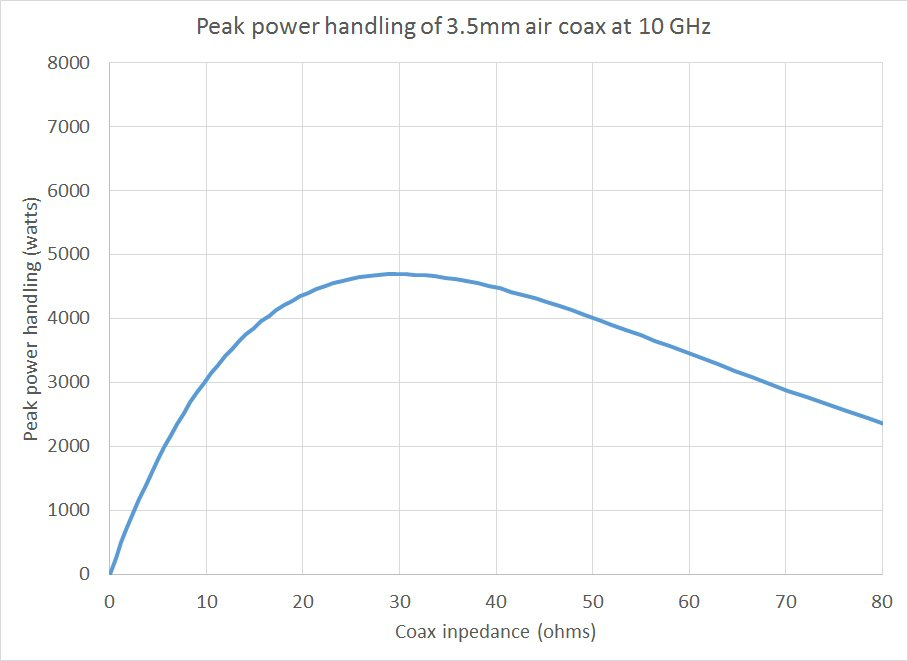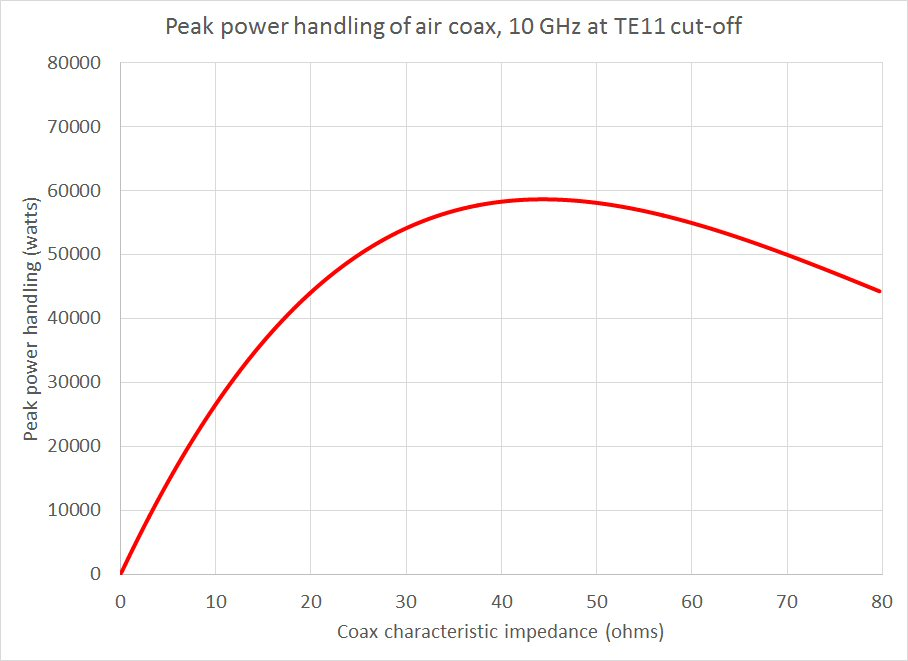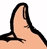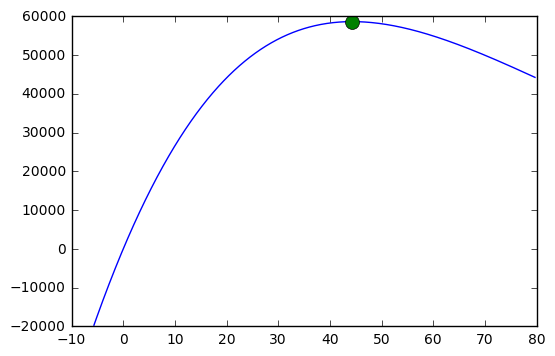# Coax absolute maximum power handling

There are plenty of resources out there that will tell you that maximum peak power handling of coax occurs at an 30 ohms impedance of air-filled coax, we might have mentioned this a time or two. This rule is true for coax that operates below the cut-off for TE11 mode. Suppose you are operating very close to the cut-off of the unwanted TE11 mode. Heck, let's assume you want to operate exactly at TE11 cut-off.  TE11 cuts off when (b+a)*pi is equal to operating wavelength.  To cut to the punch line, at TE11 cut-off, ~44 ohms carries the most power. You can find this fun fact and many more in Introduction to Microwaves by Gershon J. Wheeler, dating back to 1963.  Depending on your point of view, this is useless trivia, or a critically important information.

We decided to solve coax equations to show how 44 ohms (in air) can provide the highest power handling. We got stuck with trying to take a derivative of a messy equation, so we solved numerically using Excel.  Then for fun, we asked our good friend Alex did some math for us. There are two solutions on this page, and they even agree!

For review, let's first look at the peak-power handling curve for air coax at 10 GHz (actually, frequency does not play a part in this calculation, so long as we stay below TE11 cutoff). We chose the outer conductor diameter (3.5mm) and varied the inner conductor diameter to plot impedance versus power handling. We assumed 1,000,000 volts per meter critical field strength. The math says it is good for 4,994 watts peak, at exactly 30 ohms (this is where a/b=e/2, where "e" is Euler's constant, the base of natural logarithms). The curve needs to derated for temperature, altitude and load VSWR in real applications. Before you get excited that you could pass thousands of watts through air-coax, keep in mind that average power handling of a 3.5mm precision connector is only ~100 watts at X-band. The center-conductor is thermally isolated cannot take much heat before it starts melting its suspension donuts.At the peak power handling point for 3.5mm air coax below TE11 cutoff, the critical data are:

a=2.123mm

b=3.5mm

Z0 at peak power=30 ohms

Peak power=4,994 watts

## Numerical solution at TE11 cutoff

Here's how we approached the problem... the TE11 cutoff solution starts by assuming a frequency (10 GHz in this example), varying the inner diameter "a" from 2mm to 5mm (it took some miscalculations before the best range was established), calculating the outer diameter "b" for the condition that the coax is at TE11 cutoff, then calcuating impedance and power handling and looking for the maximum. Below is a curve showing peak power handling of coax at TE11 cutoff at 10 GHz. Note that the Y axis increments are 10X larger than on the previous plot.By making very small increments in "a" (we chose 1um steps), and employing the Excel "max" function, we arrived at peak power handling of 58,669 watts at an impedance of 44.327 ohms. At 10 GHz and operating at TE11 cutoff, the critical data are:

a=3.087mm

b=6.462mm

Z0 at peak power=44.327 ohms

Peak power=58,669 watts

Thus, power handling can be theoretically improved by more than 10X compared to 3.5mm coax at 10 GHz, if you operate on the edge of TE11. The reason for much higher power handling compared to 3.5mm coax is that that the TE11-sized conductors are much larger in diameter.

## Closed-form solution at TE11 cutoff

Alex used Wolfram to do the computation, expressing power handling in terms of "a" like the numerical solution above, then taking the derivative with regard to 'a' and setting it to zero to find the maximum value. In order to solve for that derivative, Alex used a special function called Lambert W. If you are not familiar with Lambert W, you are in good company. Support small business, visit 810Labs' website and bring Alex any microwave analysis problems that you don't have the resources to solve!

Alex came up with 44.330 ohms, we came up with 44.327 ohms, a small error on our part but no one should be worrying about fractions of an ohm in coax impedance in real life. It is nice to see the two solutions agree!

Before we present Alex's math, let's release a new Microwaves101 Rule of Thumb #124:For air coax operating at the onset of TE11, maximum peak power handling occurs at an impedance of ~44 ohms (more exactly, 44.327 ohms). At TE11 onset and 44 ohms, you can't get higher peak power handling unless you introduce a dielectric, which will increase loss.

In :
from pylab import *
from scipy.special import lambertw as W
%matplotlib inline

Ed = 1000#V/mm
f = 10 #Ghz
er=1
lam = 30#mm

def z0(a):
return 60/sqrt(er)*log(lam/(a*pi)-1)


express $Z_0$ in terms of $a$ and $\lambda$, since $b$ is a derived quantity and we are implicitly setting $\lambda = \lambda_c$

$$Z_0 = \ln{(\frac{\lambda}{a \pi}-1)}$$

Peak voltage can be expressed in $Z_0$ $$V_p = E_d a (\frac{\sqrt{\epsilon}}{60} Z_0)$$ $P_{max}$ can be expressed as

$$P_{max} = \rho a^2 Z_0 = \rho a^2 \ln{(\frac{\lambda}{a \pi}-1)}$$

where $\rho$ is a bunch of scalars which dont matter. find max by taking the derivative, set to 0,

$$\frac{\partial P_{max}}{\partial a} = \frac{a \lambda}{\pi a -\lambda} + 2 a\ln{(\frac{\lambda/\pi-a}{a})}=0$$

which is a little odd. solving this for $a$ yeilds

$$a = \frac{\lambda }{\pi e^{W(1/(2 \sqrt{e}) + 1/2} + \pi }$$
In :
a_max = lam/(pi* e**(W(1/(2 *sqrt(e))).real + 1/2) + pi)
a_max

Out:
3.0868955195491612

plugging into $Z_0$ expression, gives your answer

In :
z0(a_max)

Out:
44.330101867896481

And we can plot it if you like

In :
def P_max(a):
return (Ed*a*sqrt(er)/60)**2 * z0(a)/ 2

a = linspace(2, 5,1001)

plot(z0(a),P_max(a))
plot(z0(a_max),P_max(a_max), marker='o', markersize=10)

Out:
[<matplotlib.lines.Line2D at 0x7f813c4df320>]In [ ]:

Author : Unknown Editor